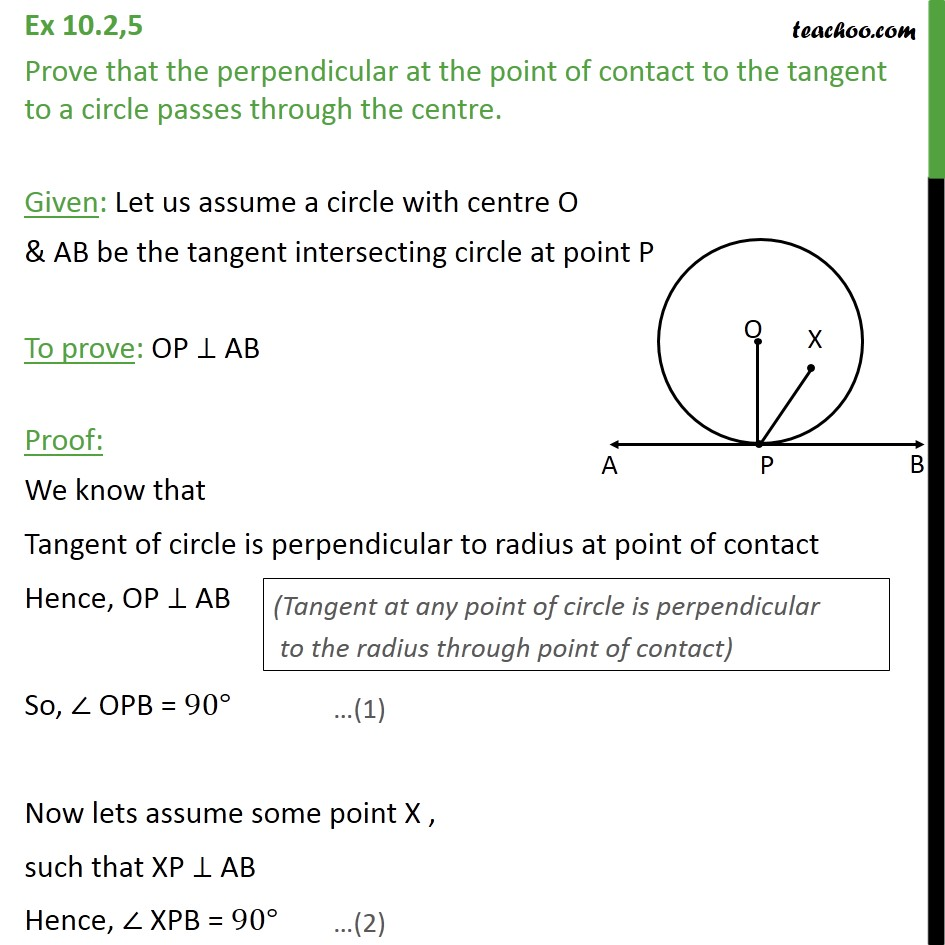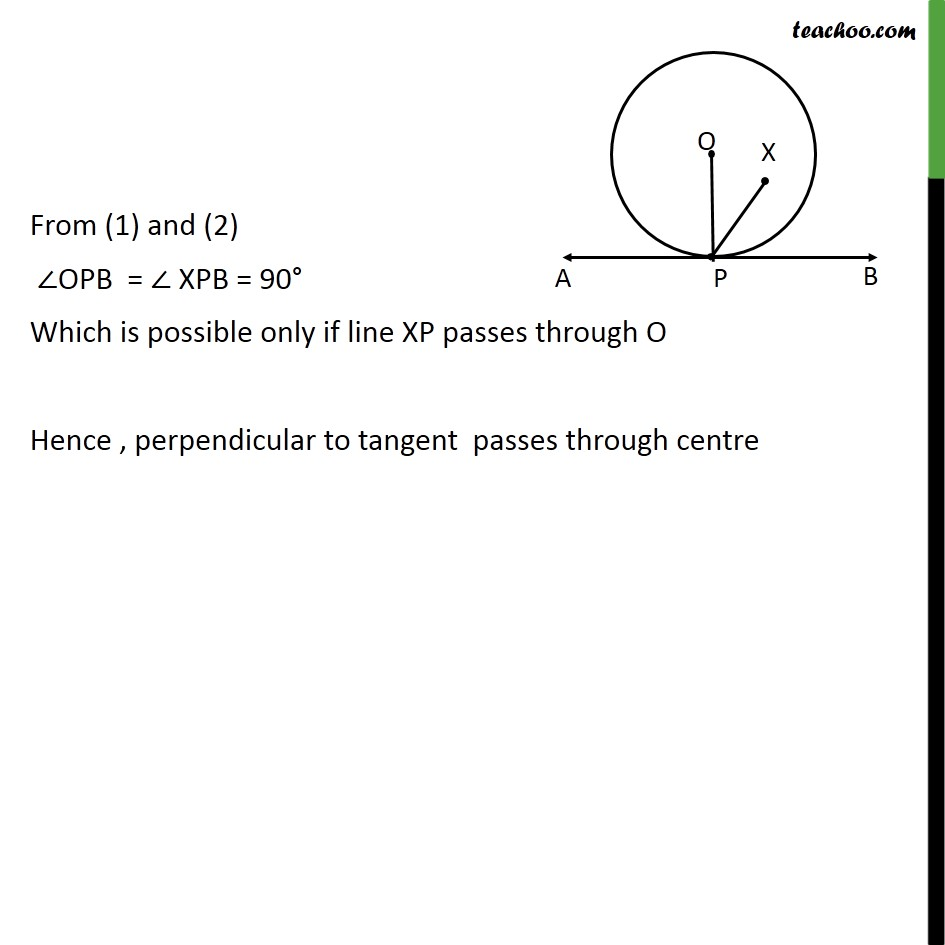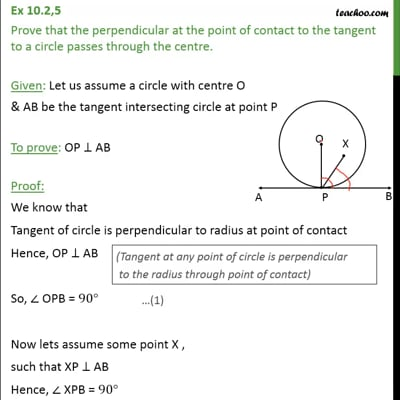Ex 10.2

Chapter 10 Class 10 Circles (Term 2)
Serial order wiseThis video is only available for Teachoo black users

### Transcript

Ex 10.2,5 Prove that the perpendicular at the point of contact to the tangent to a circle passes through the centre. Given: Let us assume a circle with centre O & AB be the tangent intersecting circle at point P To prove: OP AB Proof: We know that Tangent of circle is perpendicular to radius at point of contact Hence, OP AB So, OPB = 90 Now lets assume some point X , such that XP AB Hence, XPB = 90 From (1) and (2) OPB = XPB = 90 Which is possible only if line XP passes through O Hence , perpendicular to tangent passes through centre